# Python数据处理入门教程！

Datawhale干货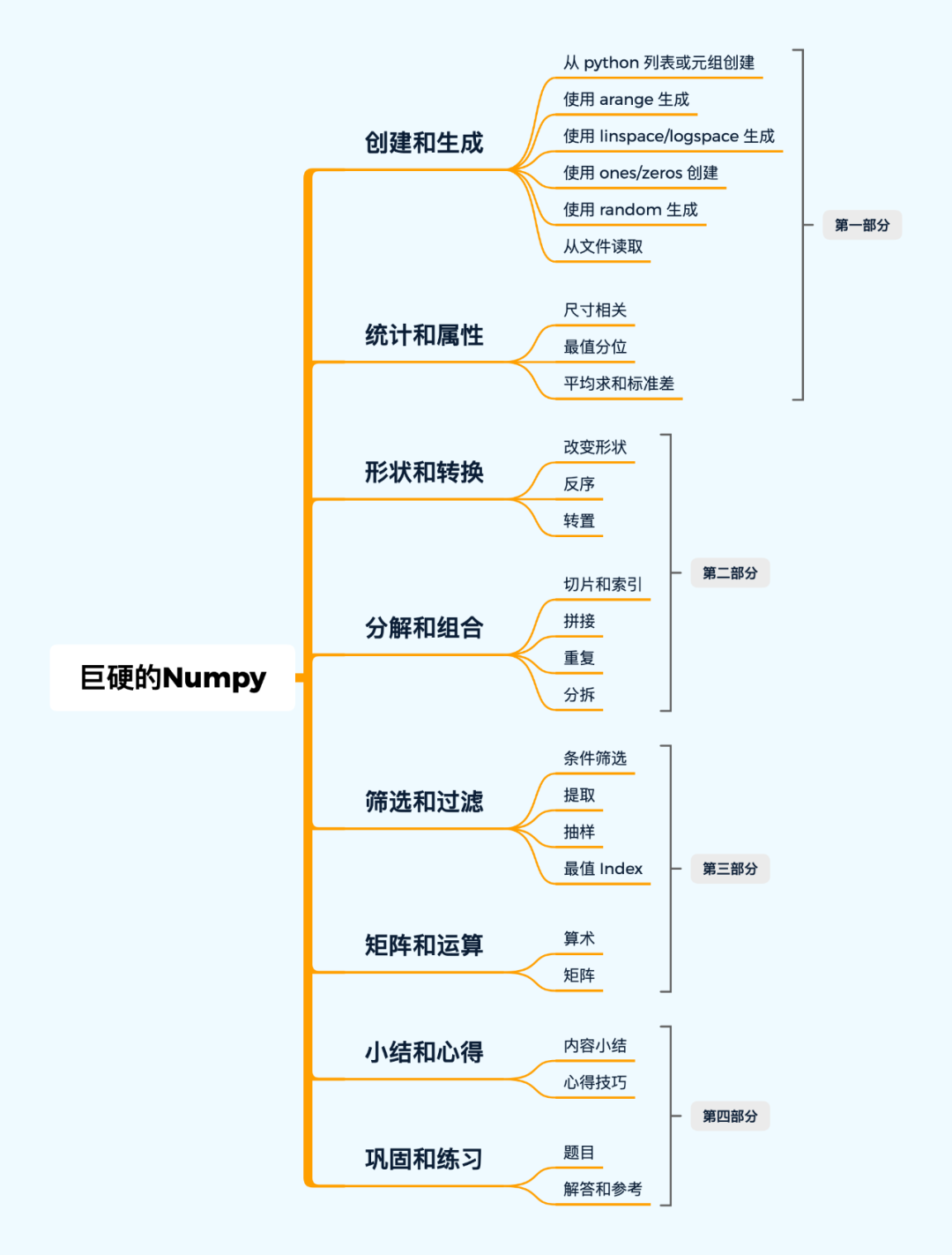· 偏实用高频 API
· 展示实际用法
· 简单直接

``````# 导入 library
import numpy as np
# 画图工具
import matplotlib.pyplot as plt
``````

## 创建和生成

• 使用列表或元组
• 使用 arange
• 使用 linspace/logspace
• 使用 ones/zeros
• 使用 random
• 从文件读取

### 从 python 列表或元组创建

⭐⭐ 重点掌握传入 list 创建一个 array 即可： `np.array(list)`

⚠️ 需要注意的是：「数据类型」。如果您足够仔细的话，可以发现下面第二组代码第 2 个数字是「小数」（注：Python 中 1. == 1.0），而 array 是要保证每个元素类型相同的，所以会帮您把 array 转为一个 float 的类型。

``````# 一个 list
np.array([1,2,3])
``````
``````array([1, 2, 3])
``````
``````# 二维（多维类似）
# 注意，有一个小数哦
np.array([[1, 2., 3], [4, 5, 6]])
``````
``````array([[1., 2., 3.],
[4., 5., 6.]])
``````
``````# 您也可以指定数据类型
np.array([1, 2, 3], dtype=np.float16)
``````
``````array([1., 2., 3.], dtype=float16)
``````
``````# 如果指定了 dtype，输入的值都会被转为对应的类型，而且不会四舍五入
lst = [
[1, 2, 3],
[4, 5, 6.8]
]
np.array(lst, dtype=np.int32)
``````
``````array([[1, 2, 3],
[4, 5, 6]], dtype=int32)
``````
``````# 一个 tuple
np.array((1.1, 2.2))
``````
``````array([1.1, 2.2])
``````
``````# tuple，一般用 list 就好，不需要使用 tuple
np.array([(1.1, 2.2, 3.3), (4.4, 5.5, 6.6)])
``````
``````array([[1.1, 2.2, 3.3],
[4.4, 5.5, 6.6]])
``````
``````# 转换而不是上面的创建，其实是类似的，无须过于纠结
np.asarray((1,2,3))
``````
``````array([1, 2, 3])
``````
``````np.asarray(([1., 2., 3.], (4., 5., 6.)))
``````
``````array([[1., 2., 3.],
[4., 5., 6.]])
``````

### 使用 arange 生成

⭐⭐

range 是 Python 内置的整数序列生成器，arange 是 numpy 的，效果类似，会生成一维的向量。我们偶尔会需要使用这种方式来构造 array，比如：

• 需要创建一个连续一维向量作为输入（比如编码位置时可以使用）
• 需要观察筛选、抽样的结果时，有序的 array 一般更加容易观察

⚠️ 需要注意的是：在 `reshape` 时，目标的 shape 需要的元素数量一定要和原始的元素数量相等。

``````np.arange(12).reshape(3, 4)
``````
``````array([[ 0,  1,  2,  3],
[ 4,  5,  6,  7],
[ 8,  9, 10, 11]])
``````
``````# 注意，是小数哦
np.arange(12.0).reshape(4, 3)
``````
``````array([[ 0.,  1.,  2.],
[ 3.,  4.,  5.],
[ 6.,  7.,  8.],
[ 9., 10., 11.]])
``````
``````np.arange(100, 124, 2).reshape(3, 2, 2
``````
``````array([[[100, 102],
[104, 106]],

[[108, 110],
[112, 114]],

[[116, 118],
[120, 122]]])
``````
``````# shape size 相乘要和生成的元素数量一致
np.arange(100., 124., 2).reshape(2,3,4)
``````
``````
ValueError: cannot reshape array of size 12 into shape (2,3,4)
``````

### 使用 linspace/logspace 生成

⭐⭐⭐

OK，这是我们遇到的第一个比较重要的 API，前者需要传入 3 个参数：开头，结尾，数量；后者需要额外传入一个 base，它默认是 10。

⚠️ 需要注意的是：第三个参数并 不是步长。

`np.linspace`

``````# 线性
np.linspace(0, 9, 10).reshape(2, 5)
``````
``````array([[0., 1., 2., 3., 4.],
[5., 6., 7., 8., 9.]])
``````
``````np.linspace(0, 9, 6).reshape(2, 3)
``````
``````array([[0. , 1.8, 3.6],
[5.4, 7.2, 9. ]])
``````
``````# 指数 base 默认为 10
np.logspace(0, 9, 6, base=np.e).reshape(2, 3)
``````
``````array([[1.00000000e+00, 6.04964746e+00, 3.65982344e+01],
[2.21406416e+02, 1.33943076e+03, 8.10308393e+03]])
``````
``````# _ 表示上（最近）一个输出
# logspace 结果 log 后就是上面 linspace 的结果
np.log(_)
``````
``````array([[0. , 1.8, 3.6],
[5.4, 7.2, 9. ]])
``````

``````N = 20
x = np.arange(N)
y1 = np.linspace(0, 10, N) * 100
y2 = np.logspace(0, 10, N, base=2)

plt.plot(x, y2, '*');
plt.plot(x, y1, 'o');
``````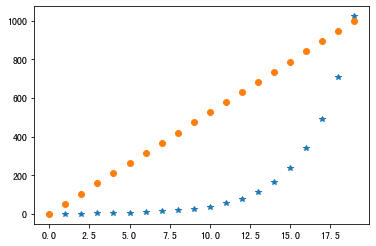``````# 检查每个元素是否为 True
# base 的 指数为 linspace 得到的就是 logspace
np.alltrue(2 ** np.linspace(0, 10, N)  == y2)
``````
``````True
⚠️ 补充：关于 array 的条件判断
``````
``````# 不能直接用 if 判断 array 是否符合某个条件
arr = np.array([1, 2, 3])
cond1 = arr > 2
cond1
``````
``````array([False, False,  True])
``````
``````if cond1:
print("这不行")
``````
``````      2     print("这不行")

ValueError: The truth value of an array with more than one element is ambiguous. Use a.any() or a.all()
``````
``````# 即便你全是 True 它也不行
arr = np.array([1, 2, 3])
cond2 = arr > 0
cond2
``````
``````array([ True,  True,  True])
``````
``````if cond2:
print("这还不行")
``````
``````      2     print("这还不行")

ValueError: The truth value of an array with more than one element is ambiguous. Use a.any() or a.all()
``````
``````# 咱们只能用 any 或 all，这个很容易犯错，请务必注意。
if cond1.any():
print("只要有一个为True就可以，所以——我可以")
``````
``````只要有一个为True就可以，所以——我可以
``````
``````if cond2.all():
print("所有值为True才可以，我正好这样")
``````
``````所有值为True才可以，我正好这样
``````

### 使用 ones/zeros 创建

⚠️ 需要注意的是：创建出来的 array 默认是 float 类型。

``````np.ones(3)
``````
``````array([1., 1., 1.])
``````
``````np.ones((2, 3))
``````
``````array([[1., 1., 1.],
[1., 1., 1.]])
``````
``````np.zeros((2,3,4))
``````
``````array([[[0., 0., 0., 0.],
[0., 0., 0., 0.],
[0., 0., 0., 0.]],

[[0., 0., 0., 0.],
[0., 0., 0., 0.],
[0., 0., 0., 0.]]])
``````
``````# 像给定向量那样的 0 向量（ones_like 是 1 向量）
np.zeros_like(np.ones((2,3,3)))
``````
``````array([[[0., 0., 0.],
[0., 0., 0.],
[0., 0., 0.]],

[[0., 0., 0.],
[0., 0., 0.],
[0., 0., 0.]]])
``````

### 使用 random 生成

⭐⭐⭐⭐⭐

⚠️ 需要注意的是：这里我们统一推荐使用新的 API 方式创建，即通过 `np.random.default_rng()` 先生成 `Generator`，然后再在此基础上生成各种分布的数据（记忆更加简便清晰）。不过我们依然会介绍就的 API 用法，因为很多代码中使用的还是旧的，您可以混个眼熟。

``````# 0-1 连续均匀分布
np.random.rand(2, 3)
``````
``````array([[0.42508994, 0.5842191 , 0.09248675],
[0.656858  , 0.88171822, 0.81744539]])
``````
``````# 单个数
np.random.rand()
``````
``````0.29322641374172986
``````
``````# 0-1 连续均匀分布
np.random.random((3, 2))
``````
``````array([[0.17586271, 0.5061715 ],
[0.14594537, 0.34365713],
[0.28714656, 0.40508807]])
``````
``````# 指定上下界的连续均匀分布
np.random.uniform(-1, 1, (2, 3))
``````
``````array([[ 0.66638982, -0.65327069, -0.21787878],
[-0.63552782,  0.51072282, -0.14968825]])
``````
``````# 上面两个的区别是 shape 的输入方式不同，无伤大雅了
# 不过从 1.17 版本后推荐这样使用（以后大家可以用新的方法）
# rng 是个 Generator，可用于生成各种分布
rng = np.random.default_rng(42)
rng
``````
``````Generator(PCG64) at 0x111B5C5E0
``````
``````# 推荐的连续均匀分布用法
rng.random((2, 3))
``````
``````array([[0.77395605, 0.43887844, 0.85859792],
[0.69736803, 0.09417735, 0.97562235]])
``````
``````# 可以指定上下界，所以更加推荐这种用法
rng.uniform(0, 1, (2, 3))
``````
``````array([[0.47673156, 0.59702442, 0.63523558],
[0.68631534, 0.77560864, 0.05803685]])
``````
``````# 随机整数（离散均匀分布），不超过给定的值（10）
np.random.randint(10, size=2)
``````
``````array([6, 3])
``````
``````# 随机整数（离散均匀分布），指定上下界和 shape
np.random.randint(0, 10, (2, 3))
``````
``````array([[8, 6, 1],
[3, 8, 1]])
``````
``````# 上面推荐的方法，指定大小和上界
rng.integers(10, size=2)
``````
``````array([9, 7])
``````
``````# 上面推荐的方法，指定上下界
rng.integers(0, 10, (2, 3))
``````
``````array([[5, 9, 1],
[8, 5, 7]])
``````
``````# 标准正态分布
np.random.randn(2, 4)
``````
``````array([[-0.61241167, -0.55218849, -0.50470617, -1.35613877],
[-1.34665975, -0.74064846, -2.5181665 ,  0.66866357]])
``````
``````# 上面推荐的标准正态分布用法
rng.standard_normal((2, 4))
``````
``````array([[ 0.09130331,  1.06124845, -0.79376776, -0.7004211 ],
[ 0.71545457,  1.24926923, -1.22117522,  1.23336317]])
``````
``````# 高斯分布
np.random.normal(0, 1, (3, 5))
``````
``````array([[ 0.30037773, -0.17462372,  0.23898533,  1.23235421,  0.90514996],
[ 0.90269753, -0.5679421 ,  0.8769029 ,  0.81726869, -0.59442623],
[ 0.31453468, -0.18190156, -2.95932929, -0.07164822, -0.23622439]])
``````
``````# 上面推荐的高斯分布用法
rng.normal(0, 1, (3, 5))
``````
``````array([[ 2.20602146, -2.17590933,  0.80605092, -1.75363919,  0.08712213],
[ 0.33164095,  0.33921626,  0.45251278, -0.03281331, -0.74066207],
[-0.61835785, -0.56459129,  0.37724436, -0.81295739,  0.12044035]])
``````

``````rng = np.random.default_rng(42)
``````
``````# 离散均匀分布
rng.integers(low=0, high=10, size=5)
``````
``````array([0, 7, 6, 4, 4])
``````
``````# 连续均匀分布
rng.uniform(low=0, high=10, size=5)
``````
``````array([6.97368029, 0.94177348, 9.75622352, 7.61139702, 7.86064305])
``````
``````# 正态（高斯）分布
rng.normal(loc=0.0, scale=1.0, size=(2, 3))
``````
``````array([[-0.01680116, -0.85304393,  0.87939797],
[ 0.77779194,  0.0660307 ,  1.12724121]])
``````

### 从文件读取

[En]

This section is mainly used to load stored weight parameters or preprocessed data sets, which is sometimes more convenient, such as loading trained model parameters into memory to provide reasoning services, or storing preprocessed data that takes a long time directly, and does not need to be reprocessed in many experiments.

⚠️ 需要注意的是：存储时不需要写文件名后缀，会自动添加。

``````# 直接将给定矩阵存为 a.npy
np.save('./data/a', np.array([[1, 2, 3], [4, 5, 6]]))
``````
``````# 可以将多个矩阵存在一起，名为 `b.npz`
np.savez("./data/b", a=np.arange(12).reshape(3, 4), b=np.arange(12.).reshape(4, 3))
``````
``````# 和上一个一样，只是压缩了
np.savez_compressed("./data/c", a=np.arange(12).reshape(3, 4), b=np.arange(12.).reshape(4, 3))
``````
``````# 加载单个 array
``````
``````array([[1, 2, 3],
[4, 5, 6]])
``````
``````# 加载多个，可以像字典那样取出对应的 array
``````
``````arr["a"]
``````
``````array([[ 0,  1,  2,  3],
[ 4,  5,  6,  7],
[ 8,  9, 10, 11]])
``````
``````arr["b"]
``````
``````array([[ 0.,  1.,  2.],
[ 3.,  4.,  5.],
[ 6.,  7.,  8.],
[ 9., 10., 11.]])
``````
``````# 后缀都一样，你干脆当它和上面的没区别即可
``````
``````arr["b"]
``````
``````array([[ 0.,  1.,  2.],
[ 3.,  4.,  5.],
[ 6.,  7.,  8.],
[ 9., 10., 11.]])
``````

## 统计和属性

• 尺寸相关
• 最大、最小、中位、分位值
• 平均、求和、标准差等

[En]

The content of this section is very simple, you only need to pay special attention to (remember) two important features:

• 按维度（指定 axis）求结果。一般0表示列1表示行，可以用「 沿着行/列操作」这样理解，不确定时可以拿个例子试一下。
• 计算后保持维度（ `keepdims=True`

``````#  先创建一个 Generator
rng = np.random.default_rng(seed=42)
#  再生成一个均匀分布
arr = rng.uniform(0, 1, (3, 4))
arr
``````
``````array([[0.77395605, 0.43887844, 0.85859792, 0.69736803],
[0.09417735, 0.97562235, 0.7611397 , 0.78606431],
[0.12811363, 0.45038594, 0.37079802, 0.92676499]])
``````

### 尺寸相关

⭐⭐

⚠️ 需要注意的是：size 不是 shape，ndim 表示有几个维度。

``````# 维度，array 是二维的（两个维度）
arr.ndim
``````
``````2
``````

#### `np.shape`

``````# 形状，返回一个 Tuple
arr.shape
``````
``````(3, 4)
``````
``````# 数据量
arr.size
``````
``````12
``````

### 最值分位

⭐⭐⭐

[En]

This section mainly includes: maximum, minimum, median, other quantiles, of which " * maximum and minimum * " we usually use the most.

⚠️ 需要注意的是：分位数可以是 0-1 的任意小数（表示对应分位），而且分位数并不一定在原始的 array 中。

``````arr
``````
``````array([[0.77395605, 0.43887844, 0.85859792, 0.69736803],
[0.09417735, 0.97562235, 0.7611397 , 0.78606431],
[0.12811363, 0.45038594, 0.37079802, 0.92676499]])
``````
``````# 所有元素中最大的
arr.max()
``````
``````0.9756223516367559
``````

#### `np.max/min`

``````# 按维度（列）最大值
arr.max(axis=0)
``````
``````array([0.77395605, 0.97562235, 0.85859792, 0.92676499])
``````
``````# 同理，按行
arr.max(axis=1)
``````
``````array([0.85859792, 0.97562235, 0.92676499])
``````
``````# 是否保持原来的维度
# 这个需要特别注意下，很多深度学习模型中都需要保持原有的维度进行后续计算
# shape 是 (3,1)，array 的 shape 是 (3,4)，按行，同时保持了行的维度
arr.min(axis=1, keepdims=True)
``````
``````array([[0.43887844],
[0.09417735],
[0.12811363]])
``````
``````# 保持维度：（1，4），原始array是（3，4）
arr.min(axis=0, keepdims=True)
``````
``````array([[0.09417735, 0.43887844, 0.37079802, 0.69736803]])
``````
``````# 一维了
arr.min(axis=0, keepdims=False)
``````
``````array([0.09417735, 0.43887844, 0.37079802, 0.69736803])
``````
``````# 另一种用法，不过我们一般习惯使用上面的用法，其实两者一回事
np.amax(arr, axis=0)
``````
``````array([0.77395605, 0.97562235, 0.85859792, 0.92676499])
``````
``````# 同 amax
np.amin(arr, axis=1)
``````
``````array([0.43887844, 0.09417735, 0.12811363])
``````
``````# 中位数
# 其他用法和 max，min 是一样的
np.median(arr)
``````
``````0.7292538655248584
``````
``````# 分位数，按列取1/4数
np.quantile(arr, q=0.25, axis=0)
``````
``````array([0.11114549, 0.44463219, 0.56596886, 0.74171617])
``````
``````# 分位数，按行取 3/4，同时保持维度
np.quantile(arr, q=0.75, axis=1, keepdims=True)
``````
``````array([[0.79511652],
[0.83345382],
[0.5694807 ]])
``````
``````# 分位数，注意，分位数可以是 0-1 之间的任何数字（分位）
# 如果是 1/2 分位，那正好是中位数
np.quantile(arr, q=1/2, axis=1)
``````
``````array([0.73566204, 0.773602  , 0.41059198])
``````

### 平均求和标准差

⭐⭐⭐

[En]

This section mainly includes: average, cumulative summation, variance, standard deviation and other further statistical indicators. Among them, the most frequently used is "average".

``````arr
``````
``````array([[0.77395605, 0.43887844, 0.85859792, 0.69736803],
[0.09417735, 0.97562235, 0.7611397 , 0.78606431],
[0.12811363, 0.45038594, 0.37079802, 0.92676499]])
``````

#### `np.average`

``````# 平均值
np.average(arr)
``````
``````0.6051555606435642
``````
``````# 按维度平均（列）
np.average(arr, axis=0)
``````
``````array([0.33208234, 0.62162891, 0.66351188, 0.80339911])
``````
``````# 另一个计算平均值的 API
# 它与 average 的主要区别是，np.average 可以指定权重，即可以用于计算加权平均
# 一般建议使用 average，忘掉 mean 吧！
np.mean(arr, axis=0)
``````
``````array([0.33208234, 0.62162891, 0.66351188, 0.80339911])
``````

#### `np.sum`

``````# 求和，不多说了，类似
np.sum(arr, axis=1)
``````
``````array([2.76880044, 2.61700371, 1.87606258])
``````
``````np.sum(arr, axis=1, keepdims=True)
``````
``````array([[2.76880044],
[2.61700371],
[1.87606258]])
``````
``````# 按列累计求和
np.cumsum(arr, axis=0)
``````
``````array([[0.77395605, 0.43887844, 0.85859792, 0.69736803],
[0.8681334 , 1.41450079, 1.61973762, 1.48343233],
[0.99624703, 1.86488673, 1.99053565, 2.41019732]])
``````
``````# 按行累计求和
np.cumsum(arr, axis=1)
``````
``````array([[0.77395605, 1.21283449, 2.07143241, 2.76880044],
[0.09417735, 1.0697997 , 1.8309394 , 2.61700371],
[0.12811363, 0.57849957, 0.94929759, 1.87606258]])
``````
``````# 标准差，用法类似
np.std(arr)
``````
``````0.28783096517727075
``````
``````# 按列求标准差
np.std(arr, axis=0)
``````
``````array([0.3127589 , 0.25035525, 0.21076935, 0.09444968])
``````
``````# 方差
np.var(arr, axis=1)
``````
``````array([0.02464271, 0.1114405 , 0.0839356 ])

``````
• NumPy 教程 | 菜鸟教程
• NumPy 中文Original: https://blog.csdn.net/Datawhale/article/details/122994699
Author: Datawhale
Title: Python数据处理入门教程！

# 0 前景提要

``````PS C:\Users\xxx\Desktop\english_cx\shen_du_xue_xi\3_1> python first.py
Traceback (most recent call last):
File "first.py", line 1, in <module>
import tensorflow as tf
ModuleNotFoundError: No module named 'tensorflow'
``````

• 我已经在使用Anaconda，但是是自己新安装Visual Studio Code，没有使用Anaconda中的install下载，所选择的anaconda版本也不带把连着连接起来的配置， *期望达到在Vscode中运行anaconda中所配置的tensorflow环境

• 已经创建好一个py文件，名为 `first.py`
• tensorflow2.0 或者tensorflow2.0-Gpu
• anaconda-2019.03-py37_0
• 已经在anaconda中搭建好环境tensorflow2.0和tensorflow2.0-gpu等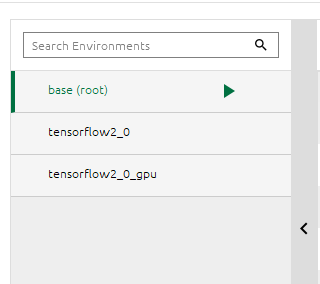# 1 安装Python插件

• 务必要在Vscode中安装python插件
在扩展中搜索python安装即可• 不然执行不了第二步

# ; 2 选择anconda中的环境# 3 运行py文件

first.py文件如下：

``````import tensorflow as tf
boston_housing = tf.keras.datasets.boston_housing

print(1+1)
``````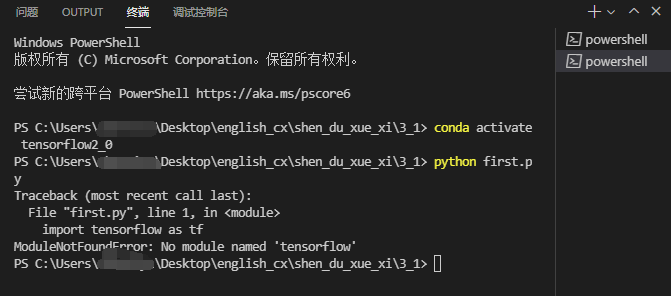1. 第一种是按图执行然后就默认启动在所需要的环境，其实是自动执行了激活环境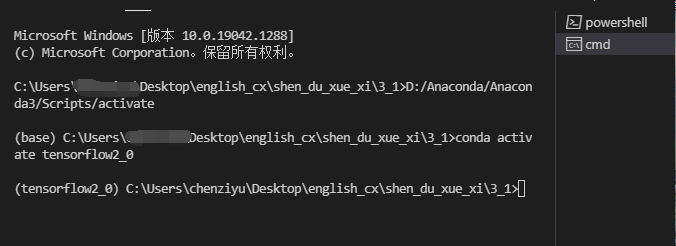然后可以直接运行py文件
``````python first.py
``````
1. 第二种是
按顺序使用如下命令即可
``````cmd
activate tensorflow2_0
python first.py
``````

# 4 参考文献

Original: https://blog.csdn.net/qq_45954434/article/details/121138853
Author: 踏破万里无云
Title: 【环境配置】在Vscode终端中使用Anaconda3中配置的环境

## 数据增强（Data Augmentation）常用方法汇总

1、数据增强的作用 1） 避免过拟合。当数据集具有某种明显的特征，例如数据集中图片基本在同一个场景中拍摄，使用Cutout方法和风格迁移变化等相关方法可避免模型学到跟目标无关的信息。 2）提升模型鲁棒...

## Tensorflow + Keras + Kaggle环境搭建+识别猫狗（For Beginners Tutorial）

TOC 序言 事实上，我也是刚刚接触Python Deep Learning and Neural Networks，作为一个Rookie，我觉的我有必要将自己的所有流程和心得体会以这样的方式记录下来...

## Docker概述（一）（标贝科技）

Docker概述（一） 顺便介绍下：我们是一家致力于智能语音交互的AI公司，我们提供了语音识别、语音合成、声纹识别、声音复刻、声音转换等技术产品供小伙伴们测试调用，感兴趣的，第三部分有详细说明！！！！...